# 【玩转数据系列十】利用阿里云机器学习在深度学习框架下实现智能图片分类

+关注继续查看

## 一、背景## 二、数据集介绍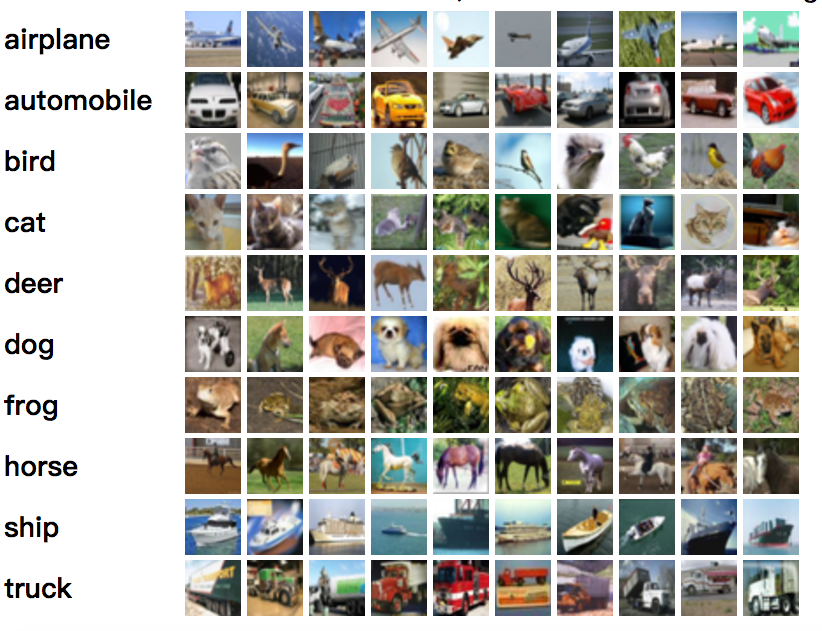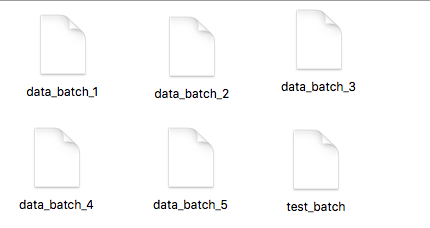## 三、数据探索流程

OSS：https://www.aliyun.com/product/oss?spm=a2c0j.103967.416540.50.KkZyBu

### 1.数据源准备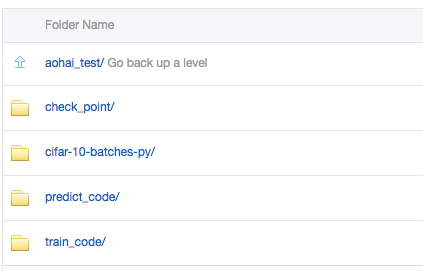• check_point:用来存放实验生成的模型
• cifar-10-batches-py：用来存放训练数据以及预测集数据，对应的是下载下来的数据源cifar-10-batcher-py文件和预测集bird_bullocks_oriole.jpg文件
• train_code:用来存放训练数据，也就是cifar_pai.py
• predict_code:用来存放cifar_predict_pai.py

### 2.配置OSS访问授权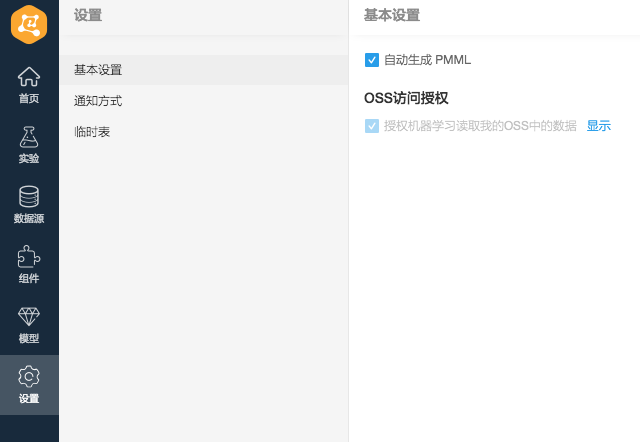### 3.模型训练

• Python代码文件：OSS中的cifar_pai.py
• 数据源目录：OSS中的cifar-10-batches-py文件夹
• 输出目录：OSS中的check_point文件夹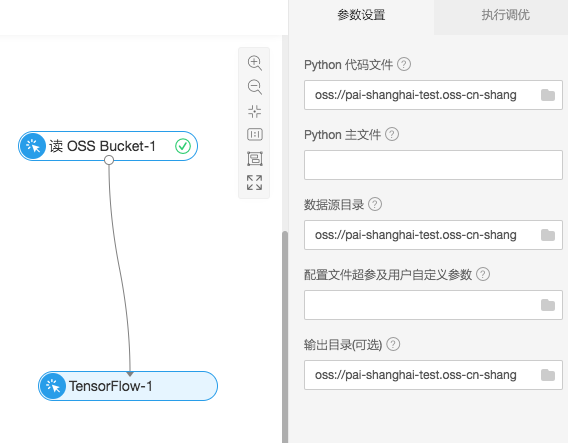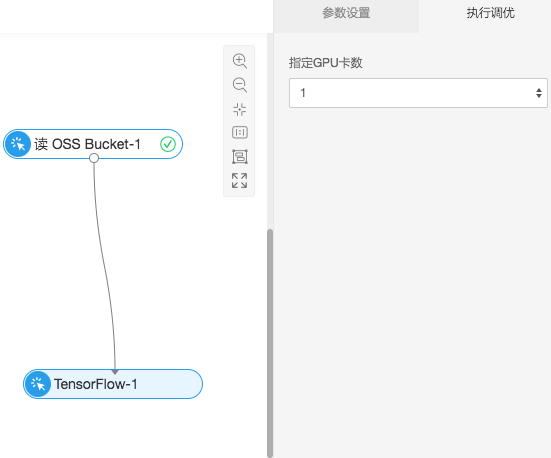### 4.模型训练代码解析

（1）构建CNN图片训练模型

network = input_data(shape=[None, 32, 32, 3],
data_preprocessing=img_prep,
data_augmentation=img_aug)
network = conv_2d(network, 32, 3, activation='relu')
network = max_pool_2d(network, 2)
network = conv_2d(network, 64, 3, activation='relu')
network = conv_2d(network, 64, 3, activation='relu')
network = max_pool_2d(network, 2)
network = fully_connected(network, 512, activation='relu')
network = dropout(network, 0.5)
network = fully_connected(network, 10, activation='softmax')
loss='categorical_crossentropy',
learning_rate=0.001)


（2）训练生成模型名为model的一系列文件，这些文件组成了TF的预测模型

    model = tflearn.DNN(network, tensorboard_verbose=0)
model.fit(X, Y, n_epoch=100, shuffle=True, validation_set=(X_test, Y_test),
show_metric=True, batch_size=96, run_id='cifar10_cnn')
model_path = os.path.join(FLAGS.checkpointDir, "model.tfl")
print(model_path)
model.save(model_path)  

### 5.查看训练过程中的日志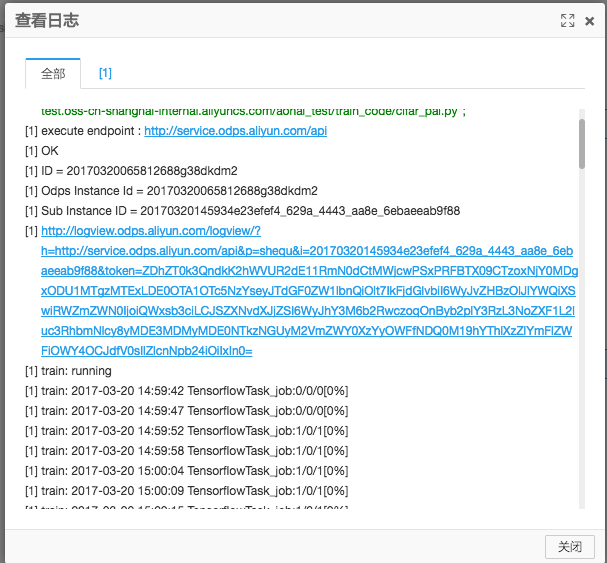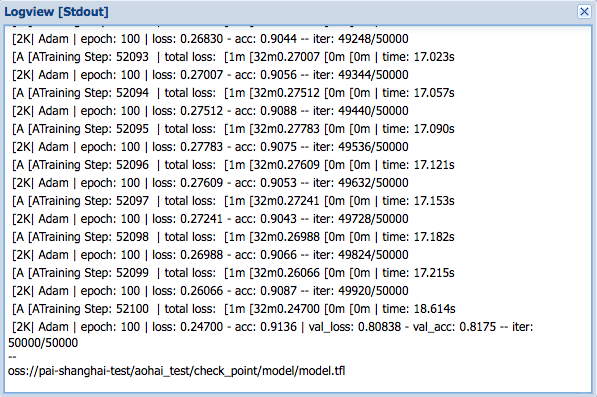### 5.结果预测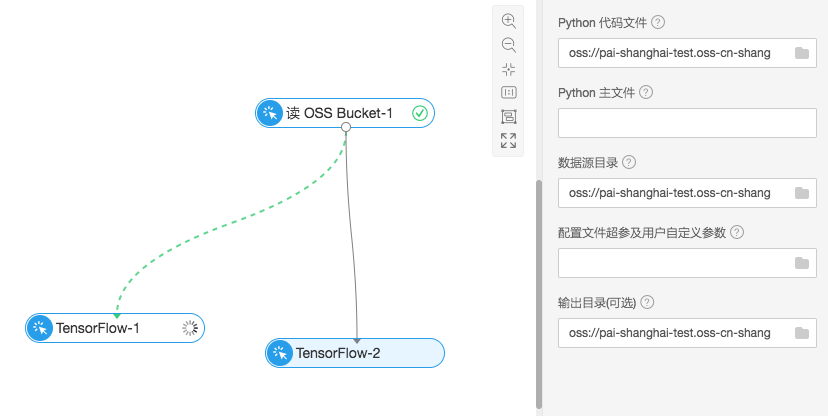• Python代码文件：OSS中的cifar_predict_pai.py
• 数据源目录：OSS中的cifar-10-batches-py文件夹,用来读取bird_mount_bluebird.jpg文件
• 输出目录：读取OSS中的check_point文件夹下模型训练生成的model.tfl模型文件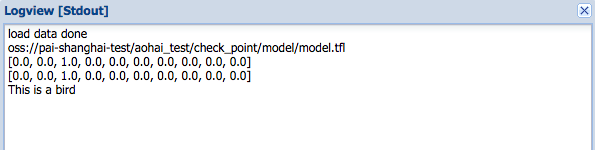### 6.预测代码数据

  predict_pic = os.path.join(FLAGS.buckets, "bird_bullocks_oriole.jpg")
file_io.write_string_to_file("bird_bullocks_oriole.jpg", img_obj)

# Scale it to 32x32
img = scipy.misc.imresize(img, (32, 32), interp="bicubic").astype(np.float32, casting='unsafe')

# Predict
prediction = model.predict([img])
print (prediction)
print (prediction)
#print (prediction.index(max(prediction)))
num=['airplane','automobile','bird','cat','deer','dog','frog','horse','ship','truck']
print ("This is a %s"%(num[prediction.index(max(prediction))]))

## 四、其它【玩转数据系列九】机器学习为您解密雾霾形成原因|
22天前
|

【阿里云OpenVI-人脸感知理解系列之人脸识别】基于Transformer的人脸识别新框架TransFace ICCV-2023论文深入解读

283 37
|
3月前
|

6025 26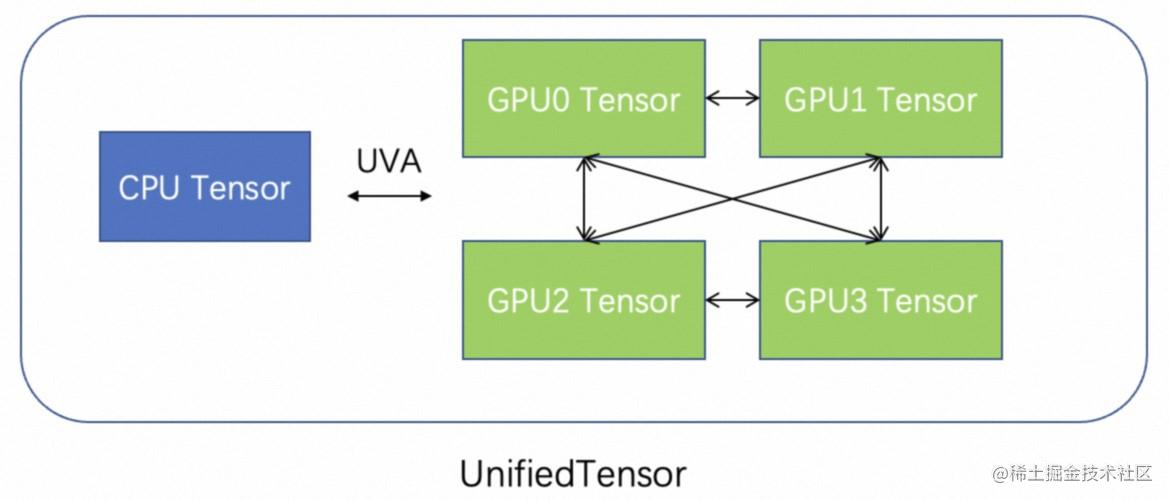|
10月前
|

333 0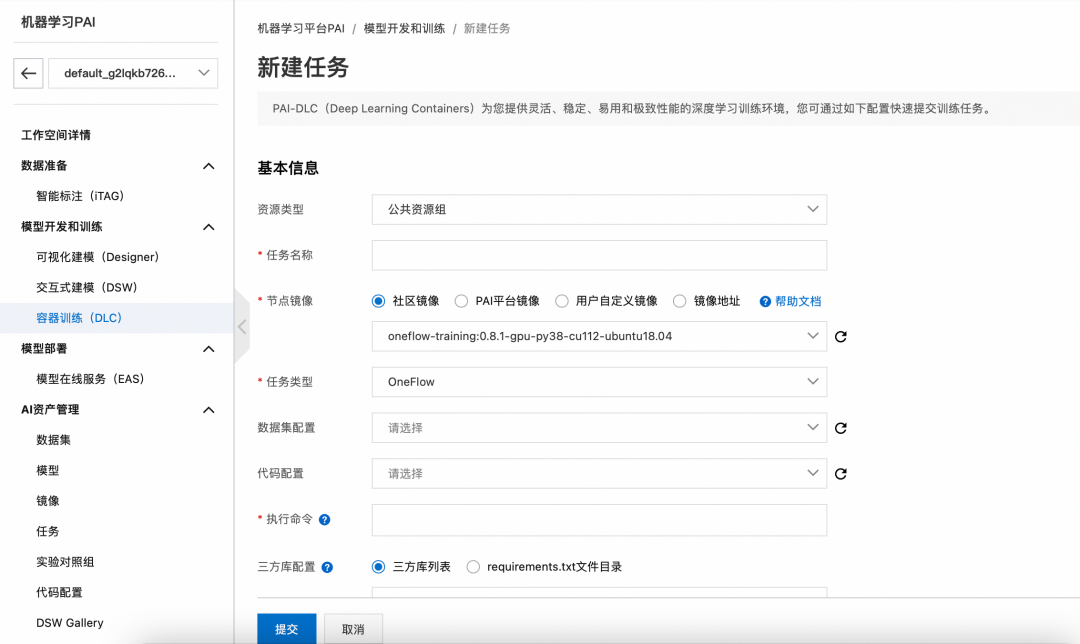|

|

125 0
|

7月10日，继阿里巴巴集团与宁波市人民政府签署战略合作框架协议仅12天后，在阿里云宁波市工业互联网中心应用推广论坛上，阿里云与宁波市江北区人民政府联合宣布，阿里云宁波市工业互联网中心正式落户宁波文创港。
473 0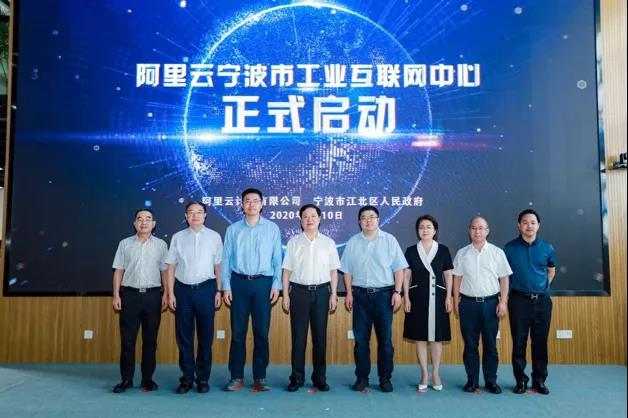|

|

2021年7月15日，阿里云与中国信息通讯院将在“2021中国互联网大会——数字化治理论坛”上， 联合发布国内业界首个《云采用框架白皮书》。
727 0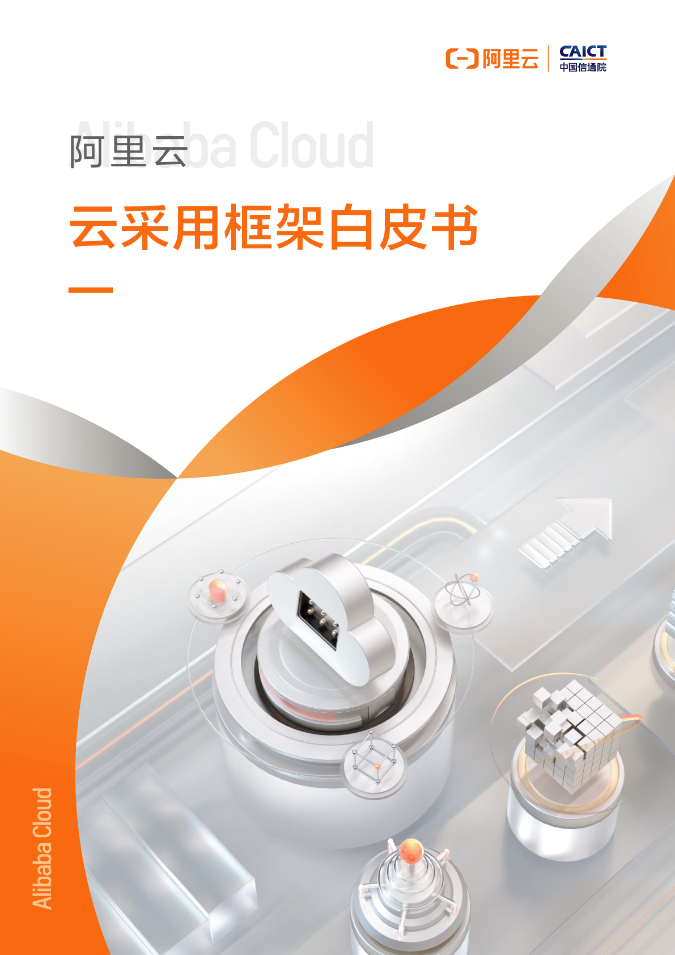|

|

ThinkPHP是一款免费、开源、快速、简单、面向对象的轻量级PHP开发框架，遵循Apache2开源协议发布，是为了敏捷Web应用开发和简化企业应用开发而诞生的。本篇教程介绍如何使用云市场镜像快速搭建ThinkPHP框架。
802 0